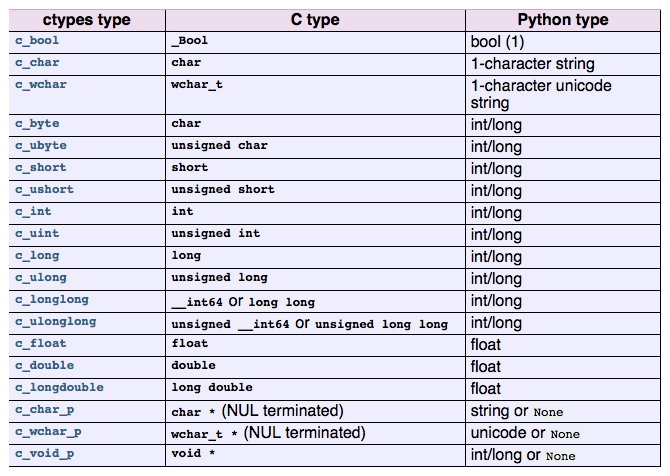# 聊聊Python ctypes 模块

=================================================================

1. ctypes 的原理以及优缺点

ctypes 有以下优点：

• Python内建，不需要单独安装
• 可以直接调用二进制的动态链接库
• 在Python一侧，不需要了解Python内部的工作方式
• 在C/C++一侧，也不需要了解Python内部的工作方式
• 对基本类型的相互映射有良好的支持

ctypes 有以下缺点：

• 平台兼容性差
• 不能够直接调用动态链接库中未经导出的函数或变量
• 对C++的支持差

2. 一个简单的例子

//great_module.c
#include <nmmintrin.h>

#ifdef _MSC_VER
#define DLL_EXPORT __declspec( dllexport )
#else
#define DLL_EXPORT
#endif

DLL_EXPORT int great_function(unsigned int n) {
return _mm_popcnt_u32(n);
}


Windows MSVC 下编译命令：（启动Visual Studio命令提示）

cl /LD great_module.c /o great_module.dll


Windows GCC、Linux、Mac OS X下编译命令相同：

gcc -fPIC -shared -msse4.2 great_module.c -o great_module.dll


from ctypes import *
great_module = cdll.LoadLibrary('./great_module.dll')
print great_module.great_function(13)


3. 类型映射：基本类型

Tm（ctypes type）、Tc（C type）、Tp （Python type） 之对应关系见下表。上面一段话比较绕。下面举个例子。

from ctypes import *
from platform import *

cdll_names = {
'Darwin' : 'libc.dylib',
'Linux'  : 'libc.so.6',
'Windows': 'msvcrt.dll'
}

clib = cdll.LoadLibrary(cdll_names[system()])
clib.printf(c_char_p("Hello %d %f"),c_int(15),c_double(2.3))


int printf (const char * format,...)


str_format = c_char_p()
int_val = c_int()
double_val = c_double()

str_format.value = "Hello %d %f"
int_val.value = 15
double_val.value = 2.3
clib.printf(str_format,int_val,double_val)


from ctypes import *
from platform import *

cdll_names = {
'Darwin' : 'libc.dylib',
'Linux'  : 'libc.so.6',
'Windows': 'msvcrt.dll'
}

clib = cdll.LoadLibrary(cdll_names[system()])
s1 = c_char_p('a')
s2 = c_char_p('b')
s3 = clib.strcat(s1,s2)
print s1.value #ab


s1 = c_char_p('a')
s3 = clib.strcat(s1,'b') # 等价于 s3 = clib.strcat(s1,c_char_p('b'))
print s1.value #ab


clib.printf(c_char_p("Hello %d %f"),15,2.3)


Traceback (most recent call last):
File "test_printf.py", line 12, in <module>
clib.printf(c_char_p("Hello %d %f"),15,2.3)
ctypes.ArgumentError: argument 3: <type 'exceptions.TypeError'>: Don't know how to convert parameter 3


4. 高级类型映射：数组

//great_module.c
#ifdef _MSC_VER
#define DLL_EXPORT __declspec( dllexport )
#else
#define DLL_EXPORT
#endif
DLL_EXPORT int array_get(int a[], int index) {
return a[index];
}


from ctypes import *
great_module = cdll.LoadLibrary('./great_module.dll')

type_int_array_10 = c_int * 10

my_array = type_int_array_10()
my_array = c_int(5)
print great_module.array_get(my_array,2)


type_int_array_10 即为创建的数组类型，如果想得到数组变量，则需要例化这个类型，即my_array。my_array的每一个成员的类型应该是 c_int，这里将它索引为2的成员赋予值 c_int(5)。当然由于隐式转换的存在，这里写 my_array = 5也完全没有问题。

from ctypes import *
from platform import *

cdll_names = {
'Darwin' : 'libc.dylib',
'Linux'  : 'libc.so.6',
'Windows': 'msvcrt.dll'
}

clib = cdll.LoadLibrary(cdll_names[system()])
s3 = clib.strcat('a','b')
print s3 # an int value like 5444948
clib.strcat.restype = c_char_p
s4 = clib.strcat('c','d')
print s4 # cd


from ctypes import *
type_int_array_10 = c_int * 10
type_int_array_10_10 = type_int_array_10 * 10
my_array = type_int_array_10_10()
my_array = 3


5. 高级类型映射：简单类型指针

ctypes 和C一样区分指针类型和指针变量。复习这两个概念：C语言里，int *是指针类型。用它声明的变量就叫指针变量。指针变量可以被赋予某个变量的地址。

type_p_int = POINTER(c_int)
v = c_int(4)
p_int = type_p_int(v)
print p_int
print p_int.contents


typedef int * type_p_int;
int v = 4;
type_p_int p = &v;
printf("%d",p);
printf("%d",*p);


from ctypes import *

type_p_int = POINTER(c_int)
v = c_int(4)
p_int = type_p_int(v)
print type(p_int)
print p_int
print p_int.contents
#-------
p_int = pointer(v)
print type(p_int)
print p_int
print p_int.contents


"#-------" 之前和之后输出的内容是一样的。

6. 高级类型映射：函数指针

1. 查看文档，将C函数指针的原型利用ctypes的CFUNCTYPE包装成ctypes函数指针类型。
2. 利用刚才得到的函数指针类型之构造函数，赋予其Python函数名，即得到函数指针变量。

qsort - C++ Reference

void qsort (void* base, size_t num, size_t size,
int (*compar)(const void*,const void*));


void qsort (int* base, size_t num, size_t size,
int (*compar)(const int*,const int*));


int compar(const int*,const int*)


CMPFUNC = CFUNCTYPE(c_int, POINTER(c_int), POINTER(c_int))


CFUNCTYPE的第一个参数是函数的返回值，函数的其他参数紧随其后。

def py_cmp_func(a, b):
print type(a)
print "py_cmp_func", a, b
return a - b


p_c_cmp_func = CMPFUNC(py_cmp_func)


from ctypes import *
from platform import *

cdll_names = {
'Darwin' : 'libc.dylib',
'Linux'  : 'libc.so.6',
'Windows': 'msvcrt.dll'
}

clib = cdll.LoadLibrary(cdll_names[system()])

CMPFUNC = CFUNCTYPE(c_int, POINTER(c_int), POINTER(c_int))

def py_cmp_func(a, b):
print type(a)
print "py_cmp_func", a, b
return a - b

type_array_5 = c_int * 5
ia = type_array_5(5, 1, 7, 33, 99)
clib.qsort(ia, len(ia), sizeof(c_int), CMPFUNC(py_cmp_func))


EnumWindows的文档见EnumWindows function (Windows)

Windows API有很多内建类型，ctypes也对应地提供了支持。代码如下：

from ctypes import *
from ctypes import wintypes

WNDENUMPROC = WINFUNCTYPE(wintypes.BOOL,
wintypes.HWND,
wintypes.LPARAM)
user32 = windll.LoadLibrary('user32.dll')

def EnumWindowsProc(hwnd, lParam):
length = user32.GetWindowTextLengthW(hwnd) + 1
buffer = create_unicode_buffer(length)
user32.GetWindowTextW(hwnd, buffer, length)
print buffer.value
return True

user32.EnumWindows(WNDENUMPROC(EnumWindowsProc), 0)


7. 其他

ctypes 还对C语言中的结构体、联合体等提供支持。这部分代码比较繁琐，可参见ctypes的文档 docs.python.org/2/libra

url：https://zhuanlan.zhihu.com/p/20152309